# Solutions to Examples of Post’s Correspondence Problem

A dataset of instances and solutions (if they exist) for Post’s correspondence problem

These were given on page 757 of A New Kind of Science (Wolfram, 2002)

## Examples

### Basic Examples

Retrieve the resource:

 In:=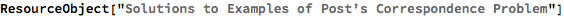Out=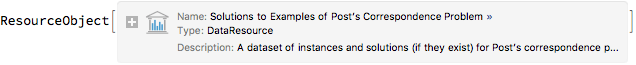Retrieve the default content:

 In:=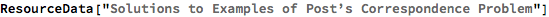Out=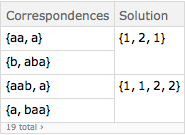Find the correspondences for the first example:

 In:=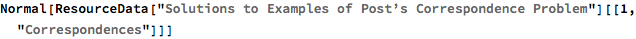Out=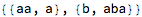Find the solution for the first example:

 In:=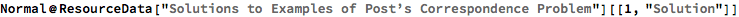Out=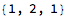Use the solution for the first problem:

 In:=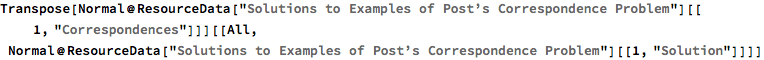Out=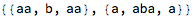Confirm that the strings are the same:

 In:=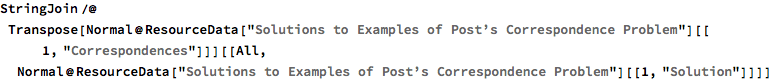Out=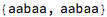In:=Out=Make a graphical version of the solution for the 10th problem:

 In:=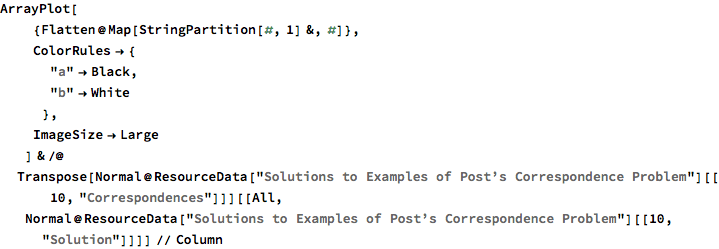Out=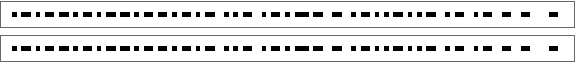Show each step of the solution for the 5th problem:

 In:=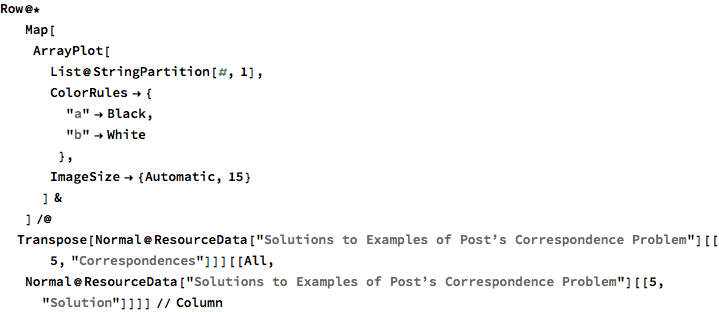Out=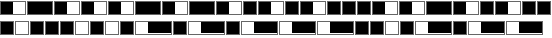Wolfram Research, "Solutions to Examples of Post’s Correspondence Problem" from the Wolfram Data Repository (2017) https://doi.org/10.24097/wolfram.68271.data